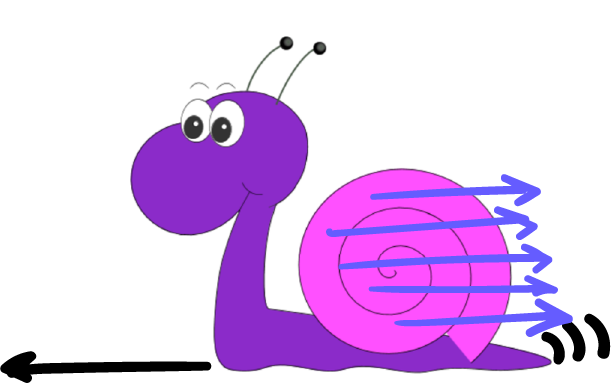# Solution: Gliding Against the Wind Puzzle

## The Puzzle:Gertie the snail is training for the Snail Olympics in a wind tunnel. If she can glide $20 cm$ in $2 \text{ hours}$ with wind assistance, and takes $3 \text{ hours}$ to cover the same distance, against the wind, how quickly can she cover the $20 \text{ cm}$ distance if there is no wind?

## The Solution:

It's very tempting to say that Gertie's average speed is $\dfrac{20 + 20}{2 + 3} = \dfrac{40}{5} = 8 \text{ cm/h}$, so she'll take $\dfrac{20}{8} = 2 \; \dfrac{1}{2}$ hours to glide the $20$ cm distance if there's no wind. Unfortunately, this is incorrect! The problem is that she only travels for $2$ hours with assistance from the wind, and $3$ hours against the wind, so taking the average speed won't give us an accurate idea of how long Gertie would take to glide $20$ cm without any wind at all. We need to be a bit cleverer and work out the distances she would travel in equal times in each direction!

What should we do? Well, we can work out how far Gertie can glide with the wind in $3$ hours. This will give us a total distance travelled in $6$ hours, which should cancel out the effects of the wind, and we can use this to find the time it will take Gertie to glide for $20$ centimetres without the wind.

If it takes Gertie $2$ hours to glide $20$ cm with the wind, she should be able to glide $1.5$ times that distance ($30 \text{ cm}$) in $3$ hours. So, her speed without any wind should be given by

$\begin{eqnarray*} \text{average speed} &= \dfrac{\text{distance travelled}}{\text{time taken}} \\ &= \dfrac{30 + 20}{3 + 3}\\ &= \dfrac{50}{6}\\ &= 8 \; \dfrac{1}{3} \text{ cm/h} \end{eqnarray*}$

We can use this speed to calculate the time taken to glide the $20$ cm distance without any wind:

$\begin{eqnarray*} \text{time} &= \dfrac{\text{distance}}{\text{speed}} \\ &= \dfrac{20}{8\;\dfrac{1}{3}}\\ &= \dfrac{60}{25}\\ &= \dfrac{12}{5}\\ &= 2.4 \text{ hours}. \end{eqnarray*}$
So, it would take Gertie $2.4$ hours to glide $20$ cm without the wind.

### Description

This series of puzzles are for Year 10 or higher students, these puzzles tests your skills and also train you with problem solving and thinking out of the box

### Audience

Year 10 students or higher

### Learning Objectives

Solving puzzles

Author: Subject Coach
You must be logged in as Student to ask a Question.